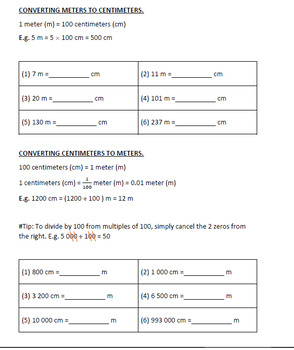# Measurement: Conversion of Kilometers, Meters and Centimeters BundleSubject
Resource Type
Format
Zip (2 MB)
Standards
\$3.49
Bundle
List Price:
\$3.88
You Save:
\$0.39
Products in this Bundle (2)
1. There are 10 worksheets that include of conversion of kilometers, meters and centimeters, including addition and subtraction of kilometers, meters and centimeters. Each worksheet can stand alone, you can choose which practice to give to your students. The questions are different from the other work
2. This is a worksheet of conversion of meters and centimeters, including addition and subtraction of meters and centimeters. 1st Practice: Converting meters to centimeters, meters to centimeters. 6 questions each. Basic level as it only deals with whole numbers. 2nd Practice: Converting meters to cent
• Bundle Description
• Standards

This bundle includes NO PREP practice for the conversion of Kilometers, Meters and Centimeters. The two worksheets, Measurement: Conversion of Meters and Centimeters & Measurement: Conversion of Kilometers, Meters and Centimeters come with answers.

Measurement: Conversion of Meters and Centimeters

1st Practice: Converting meters to centimeters, meters to centimeters. 6 questions each. Basic level as it only deals with whole numbers.

2nd Practice: Converting meters to centimeters, meters to centimeters. 6 questions each. Intermediate level as it inclusive of decimals.

3rd Practice:

Converting meters to meters and centimeters. (6 questions)

Converting meters and centimeters to meters. (4 questions)

Converting meters and centimeters to centimeters. (4 questions)

4th Practice:

Addition of meters and centimeters. (8 questions) You can choose to let your students to convert it back to cm, m or m and cm. But the answer key is in terms of m and cm.

5th Practice:

Subtraction of meters and centimeters. (8 questions) You can choose to let your students to convert it back to cm, m or m and cm. But the answer key is in terms of m and cm.

Measurement: Conversion of Kilometers, Meters and Centimeters

Practice 1: Converting kilometers to meters, meters to kilometers. 10 questions each. Basic level as it only deals with whole numbers.

Practice 2: Converting kilometers to meters, meters to kilometers. 10 questions each. Intermediate level as it inclusive of decimals.

Practice 3: Converting meters to centimeters, centimeters to meters. 10 questions each. Basic level as it only deals with whole numbers.

Practice 4: Converting meters to centimeters, centimeters to meters. 10 questions each. Intermediate level as it inclusive of decimals.

Practice 5:

Converting kilometers to kilometers and meters. (6 questions)

Converting kilometers and meters to kilometers. (4 questions)

Converting kilometers and meters to meters. (4 questions)

Practice 6:

Converting meters to meters and centimeters. (6 questions)

Converting meters and centimeters to meters. (4 questions)

Converting meters and centimeters to centimeters. (4 questions)

Practice 7:

Addition of kilometers and meters. (9 questions) You can choose to let your students to convert it back to m, km or km and cm. Answer key is in terms of m, km and m.

Practice 8:

Subtraction of kilometers and meters. (9 questions) You can choose to let your students to convert it back to m, km or km and cm. Answer key is in terms of m, km and m.

Practice 9:

Addition of meters and centimeters. (9 questions) You can choose to let your students to convert it back to cm, m or m and cm. But the answer key is in terms of cm, m and cm.

Practice 10:

Subtraction of meters and centimeters. (8 questions) You can choose to let your students to convert it back to cm, m or m and cm. But the answer key is in terms of cm, m and cm.

▼▼▼▼▼▼▼▼▼▼▼▼▼▼▼▼▼▼▼▼▼▼▼▼▼▼▼▼▼▼▼▼▼▼▼▼▼▼▼▼▼▼▼▼

You Might Also Like:

Grid Logic Puzzles Math Measurements No Prep

Circles Math Detective: The Dead Planets | Scavenger Hunt + Grid Logic Puzzles

Permission to copy for single classroom use only.

Electronic distribution limited to single classroom use only.

Not for public display

to see state-specific standards (only available in the US).
Convert among different-sized standard measurement units within a given measurement system (e.g., convert 5 cm to 0.05 m), and use these conversions in solving multi-step, real world problems.
Know relative sizes of measurement units within one system of units including km, m, cm; kg, g; lb, oz.; l, ml; hr, min, sec. Within a single system of measurement, express measurements in a larger unit in terms of a smaller unit. Record measurement equivalents in a two-column table. For example, know that 1 ft is 12 times as long as 1 in. Express the length of a 4 ft snake as 48 in. Generate a conversion table for feet and inches listing the number pairs (1, 12), (2, 24), (3, 36),...
Total Pages
N/A
Included
Teaching Duration
N/A
Report this Resource to TpT
Reported resources will be reviewed by our team. Report this resource to let us know if this resource violates TpT’s content guidelines.
• Ratings & Reviews
• Q & A

Teachers Pay Teachers is an online marketplace where teachers buy and sell original educational materials.